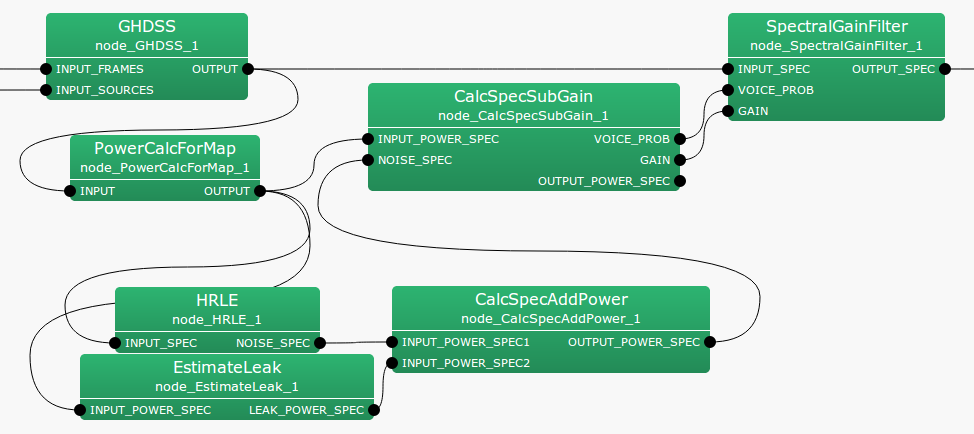## 6.3.3 CalcSpecSubGain

### 6.3.3.1 Outline of the node

This node determines an optimum gain for how much of the power spectrum of estimated noise is to be removed from a power spectrum including signals + noise. Further, it outputs the probability of speech presence (See Section 6.3.11). However, this node constantly outputs 1 as the probability of speech presence. It outputs the difference between a separated sound’s power spectrum and the estimated noise’s power spectrum.

### 6.3.3.2 Necessary file

No files are required.

### 6.3.3.3 Usage

When to use

It is used when performing noise estimation with the HRLE node.

Typical connection

Figure 6.53 shows a connection example of CalcSpecSubGain . Inputs are power spectra after separation with GHDSS and those of the noise estimated in HRLE . Its outputs connect VOICE_PROB and GAIN to SpectralGainFilter .Figure 6.53: Connection example of CalcSpecSubGain

### 6.3.3.4 Input-output and property of the node

Table 6.45: Parameters of CalcSpecSubGain
 Parameter name Type Default value Unit Description ALPHA 1.0 Gain for spectral subtraction BETA 0.0 Floor for GAIN SS_METHOD 2 Selection of power/amplitude spectra

Input

INPUT_POWER_SPEC

: Map<int, ObjectRef> type. Vector<float> type data pair of a sound source ID and a power spectrum of the separated sound.

NOISE_SPEC

: Map<int, ObjectRef> type. Vector<float> type data pair of a sound source ID and a power spectrum of the estimated sound.

Output

VOICE_PROB

: Map<int, ObjectRef> type. Vector<float> type data pair of a sound source ID and a power spectrum of the probability of speech presence.

GAIN

: Map<int, ObjectRef> type. Vector<float> type data pair of a sound source ID and a power spectrum of the optimum sound.

OUTPUT_POWER_SPEC

: Map<int, ObjectRef> type. Vector<float> type data pair of the sound source ID and the power spectrum of the separated sound with the estimated noise deducted.

Parameter

ALPHA

: float type. Gain for spectral subtraction.

BETA

: float type. Spectral floor.

SS_METHOD

: int type. Selection of power/amplitude spectra for the spectral subtraction.

### 6.3.3.5 Details of the node

This node determines an optimum gain for how much of the estimated noise’s power spectrum of the estimated noise is to be removed when a noise power spectrum is removed from a power spectrum of signals + noise. It also outputs the probability of speech presence. (See Section 6.3.11.) However, this node constantly outputs 1 as the probability of speech presence. It outputs the difference of the power spectrum of the separated sound and that of the estimated noise. Assuming that the power spectrum from which noise was is $Y_ n(k_ i)$, the power spectrum of the separated sound is $X_ n(k_ i)$ and that of the noise estimated is $N_ n(k_ i)$, the output from OUTPUT_POWER_SPEC is expressed as follows.

 $\displaystyle Y_ n(k_ i)$ $\displaystyle =$ $\displaystyle X_ n(k_ i)- N_ n(k_ i)$ (46)

Here, $n$ indicates an analysis frame number. $k_ i$ indicates a frequency index. The optimum gain $G_ n(k_ i)$ is expressed as follows.

 $\displaystyle G_ n(k_ i)$ $\displaystyle =$ $\displaystyle \left\{ \begin{array}{cr} {\rm ALPHA}\frac{Y_ n(k_ i)}{X_ n(k_ i)}, & {if~ ~ } Y_ n(k_ i)> {\rm BETA}, \\ {\rm BETA},& {if~ ~ otherwise}. \end{array} \right.$ (47)

When processing simply with $Y_ n(k_ i)$, power can become negative. The purpose of this node is to calculate a gain for removing power spectra of noise beforehand so that power cannot be negative, since it might become difficult to treat such a power spectrum in subsequent processing.